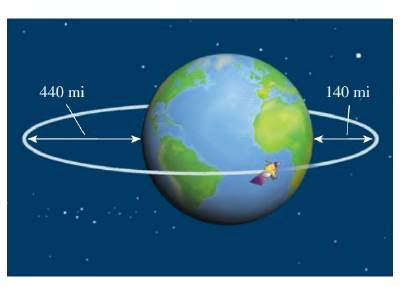Chapter 12.4, Problem 67E### Algebra and Trigonometry (MindTap ...

4th Edition
James Stewart + 2 others
ISBN: 9781305071742

#### Solutions

Chapter
Section### Algebra and Trigonometry (MindTap ...

4th Edition
James Stewart + 2 others
ISBN: 9781305071742
Textbook Problem

# Orbit of a satellite A satellite is in an elliptical orbit around the earth with the center of the earth at one focus, as shown in the figure. The height of the satellite above the earth varies between 140   mi and 440   mi . Assume that the earth is a sphere with radius 3960   mi Find an equation for the path of the satellite with the origin at the center of the earth.To determine

The equation for the path of a satellite with the origin at the center of the earth.

Explanation

Given:

Earth is in spherical shape with radius 3690mi.

Centre of the earth is (0,0).

Height of the satellite above the earth lies between 140mi and 440mi and one of its foci is at the centre of the earth.

Approach:

The general equation of an ellipse is

(xh)2a2+(yk)2b2=1(1)

Here, (h,k) is the centre of ellipse and a, b are the length of major or minor axis.

Calculation:

Suppose that major axis of elliptical orbit is x-axis.

Then, from the given figure

2a=440+2r+140

Here, a is the length of major axis and r is the radius of the earth.

So,

2a=440+2(3960)+1402a=8500a=4250

Again from the figure, the distance between focus and one end of major axis is

aae=140+r

Here, e is the eccentricity.

So,

aae=140+3960aae=41004250ae=4100ae=150

e=1504250

Now, e is given by

e=ca

Here, c=a2b2

### Still sussing out bartleby?

Check out a sample textbook solution.

See a sample solution

#### The Solution to Your Study Problems

Bartleby provides explanations to thousands of textbook problems written by our experts, many with advanced degrees!

Get Started